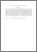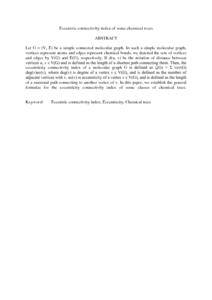# Eccentric connectivity index of some chemical trees

## Citation

Haoer, R. S. and Mohd Atan, Kamel Ariffin and Khalaf, A. M. and Md. Said, Mohamad Rushdan and Hasni, Roslan (2016) Eccentric connectivity index of some chemical trees. International Journal of Pure and Applied Mathematics, 106 (1). pp. 157-170. ISSN 1311-8080; ESSN: 1314-3395

## Abstract

Let G = (V, E) be a simple connected molecular graph. In such a simple molecular graph, vertices represent atoms and edges represent chemical bonds, we denoted the sets of vertices and edges by V(G) and E(G), respectively. If d(u, v) be the notation of distance between vertices u, v ε V(G) and is defined as the length of a shortest path connecting them. Then, the eccentricity connectivity index of a molecular graph G is defined as ζ(G) = Σ vεv(G) deg(v)ec(v), where deg(v) is degree of a vertex v ε V(G), and is defined as the number of adjacent vertices with v. ec(v) is eccentricity of a vertex v ε V(G), and is defined as the length of a maximal path connecting to another vertex of v. In this paper, we establish the general formulas for the eccentricity connectivity index of some classes of chemical trees.Preview
PDF (Abstract)
Eccentric connectivity index of some chemical trees.pdfView Item